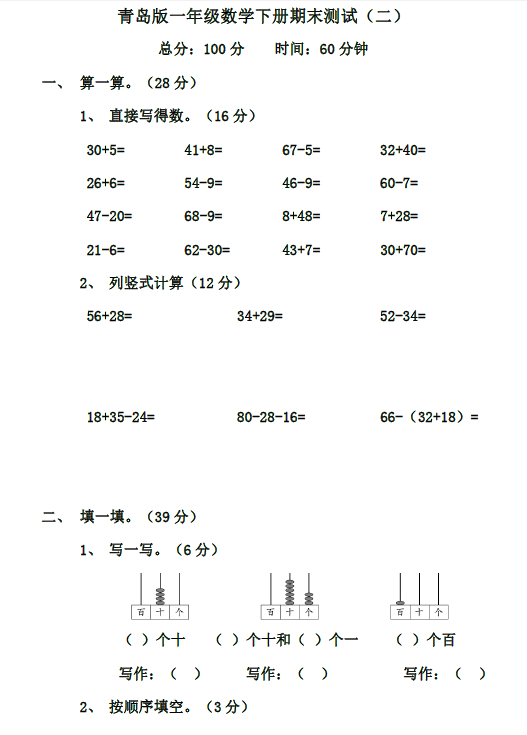1、直接写得数。（16 分）
30+5= 41+8= 67-5= 32+40=
26+6= 54-9= 46-9= 60-7=
47-20= 68-9= 8+48= 7+28=

【PDF文档6页】小学青岛版一年级数学下册期末测试（二）含答案A4电子版资料_可直接打印_会员免费下载评论信息